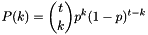#Boost C++ Libraries

...one of the most highly regarded and expertly designed C++ library projects in the world.

This is the documentation for an old version of Boost. Click here to view this page for the latest version.

## Class template binomial_distribution

boost::random::binomial_distribution

## Synopsis

```// In header: <boost/random/binomial_distribution.hpp>

template<typename IntType = int, typename RealType = double>
class binomial_distribution {
public:
// types
typedef IntType  result_type;
typedef RealType input_type;

// member classes/structs/unions

class param_type {
public:
// types
typedef binomial_distribution distribution_type;

// construct/copy/destruct
explicit param_type(IntType = 1, RealType = 0.5);

// public member functions
IntType t() const;
RealType p() const;

// friend functions
template<typename CharT, typename Traits>
friend std::basic_ostream< CharT, Traits > &
operator<<(std::basic_ostream< CharT, Traits > &, const param_type &);
template<typename CharT, typename Traits>
friend std::basic_istream< CharT, Traits > &
operator>>(std::basic_istream< CharT, Traits > &, param_type &);
friend bool operator==(const param_type &, const param_type &);
friend bool operator!=(const param_type &, const param_type &);
};

// construct/copy/destruct
explicit binomial_distribution(IntType = 1, RealType = 0.5);
explicit binomial_distribution(const param_type &);

// public member functions
template<typename URNG> IntType operator()(URNG &) const;
template<typename URNG> IntType operator()(URNG &, const param_type &) const;
IntType t() const;
RealType p() const;
IntType min() const;
IntType max() const;
param_type param() const;
void param(const param_type &);
void reset();

// friend functions
template<typename CharT, typename Traits>
friend std::basic_ostream< CharT, Traits > &
operator<<(std::basic_ostream< CharT, Traits > &,
const binomial_distribution &);
template<typename CharT, typename Traits>
friend std::basic_istream< CharT, Traits > &
operator>>(std::basic_istream< CharT, Traits > &, binomial_distribution &);
friend bool operator==(const binomial_distribution &,
const binomial_distribution &);
friend bool operator!=(const binomial_distribution &,
const binomial_distribution &);
};```

## Description

The binomial distribution is an integer valued distribution with two parameters, `t` and `p`. The values of the distribution are within the range [0,t].

The distribution function is.

The algorithm used is the BTRD algorithm described in

"The generation of binomial random variates", Wolfgang Hormann, Journal of Statistical Computation and Simulation, Volume 46, Issue 1 & 2 April 1993 , pages 101 - 110

### `binomial_distribution` public construct/copy/destruct

1. `explicit binomial_distribution(IntType t = 1, RealType p = 0.5);`

Construct a `binomial_distribution` object. `t` and `p` are the parameters of the distribution.

Requires: t >=0 && 0 <= p <= 1

2. `explicit binomial_distribution(const param_type & param);`

Construct an `binomial_distribution` object from the parameters.

### `binomial_distribution` public member functions

1. `template<typename URNG> IntType operator()(URNG & urng) const;`

Returns a random variate distributed according to the binomial distribution.

2. ```template<typename URNG>
IntType operator()(URNG & urng, const param_type & param) const;```

Returns a random variate distributed according to the binomial distribution with parameters specified by `param`.

3. `IntType t() const;`

Returns the `t` parameter of the distribution.

4. `RealType p() const;`

Returns the `p` parameter of the distribution.

5. `IntType min() const;`

Returns the smallest value that the distribution can produce.

6. `IntType max() const;`

Returns the largest value that the distribution can produce.

7. `param_type param() const;`

Returns the parameters of the distribution.

8. `void param(const param_type & param);`

Sets parameters of the distribution.

9. `void reset();`

Effects: Subsequent uses of the distribution do not depend on values produced by any engine prior to invoking reset.

### `binomial_distribution` friend functions

1. ```template<typename CharT, typename Traits>
friend std::basic_ostream< CharT, Traits > &
operator<<(std::basic_ostream< CharT, Traits > & os,
const binomial_distribution & bd);```

Writes the parameters of the distribution to a `std::ostream`.

2. ```template<typename CharT, typename Traits>
friend std::basic_istream< CharT, Traits > &
operator>>(std::basic_istream< CharT, Traits > & is,
binomial_distribution & bd);```

Reads the parameters of the distribution from a `std::istream`.

3. ```friend bool operator==(const binomial_distribution & lhs,
const binomial_distribution & rhs);```

Returns true if the two distributions will produce the same sequence of values, given equal generators.

4. ```friend bool operator!=(const binomial_distribution & lhs,
const binomial_distribution & rhs);```

Returns true if the two distributions could produce different sequences of values, given equal generators.

 Copyright © 2000-2005 Jens MaurerCopyright © 2009, 2010 Steven Watanabe Distributed under the Boost Software License, Version 1.0. (See accompanying file LICENSE_1_0.txt or copy at http://www.boost.org/LICENSE_1_0.txt)